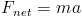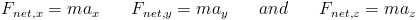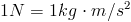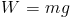# Summary of Chapter 5: Forces

Newtonian Mechanics The velocity of a particle can change when one or more forces (pushes or pulls) act upon it.

Force Forces are vector quantities (see Chapter 3:Vectors) Their magnitudes are in terms of the accelaration they would supply to a mass weight in kilograms. a force that accelerates a 1 kg body by 1 m/s2 has a magnetude of 1 Newton. Newtons are the unit of measure for forces. A force's direction is the same as the acceleration is causes. The net force on a body is the vector sum of all forces acting on the body.

Newton's First Law A body at rest remains at rest unless acted upon by an unbalanced external force.

Inertial Refrence Frames Rafrence frames in which newtoniam mechanics hold are called inertial refrence frames or inertial frames. Frefrence frames in which newtonian mechanics do not hold are called noniinertial refrence frames.

Mass Mass is a caracteristic of a body that relates the body's acceleration and the net force on that body. Mass is a scalar quantities.

Newton's Second Law The net force (Fnet) on a body with mass m is related to the bodys acceleration a by:which can also be written in component form:The Newton is the SI measurment for force:A free body diagram is a diagram in which all the external forces on a single body are drawn on a coordinate system, oriented as to simplify the solution.

Specific Forces Gravitational force (FG) on a body is a pull by another body. Usually, the second body is earth. The gravitational force is given by:Where m is the particles mass, and g is the free fall acceleration. (the free fall acceleration on earch is 9.8 m/s2)

the weight (W) of a body is hte magnitude of upward force needed to balance the gravitatioinal force on a body. Weight is given by:A normal force (FN) is the force on a body from a surface on which it presses. A normal force is always perpendicular to the surface.

A frictional force is the force on a body when a body attempts to slide along a surface. the force is always opposed to the sliding and parallel to the surface.

when a cord is under tension, each end of the cord is pulling on a body. the pull is directed parallel to the cord. On a massless cord, the tension at both ends is equal even if it runs around a massless frictionless pulley.

Newton's Third Law The force of object B on object C is equal to the force of C on B. (For every action, there is an equal and opposite reaction.)Return to top
All information and equations are taken from and credited to Jearl Walker's Fundamental Physics.